﻿ 叶片粗糙度对压气机性能影响研究 Study on the Influence of Blade Roughness on Compressor Performance

Applied Physics
Vol. 09  No. 08 ( 2019 ), Article ID: 31660 , 8 pages
10.12677/APP.2019.98043

Study on the Influence of Blade Roughness on Compressor Performance

Tao Sun1, Yan Wang2, Hanqiang Song3

1Naval Aviation Academy Fundamental College, Yantai Shandong

2The PLA Navy’s First Military Representative Office in Shenyang, Shenyang Liaoning

3The Army of Unit 92728, Shanghai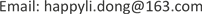Received: July 21st, 2019; accepted: August 5th, 2019; published: August 12th, 2019ABSTRACT

There are many factors that affect the performance of aero-engine, among which the change of compressor blade surface roughness caused by scaling is the representative one. The change of blade surface roughness directly affects the change of effective flow area of air passage, and then causes the change of supercharging capacity, efficiency and flow capacity. In this paper, the flow field simulation technique is used to study the performance variation of compressor under different blade roughness. The results show that when the blade roughness decreases, the compressor boost ratio and efficiency increase significantly, the shock wave intensity on the blade surface decreases, the total temperature decreases, and the overall performance of the compressor improves significantly.

Keywords:Blade, Roughness, Flow Field Simulation, Compressor Performance

1海军航空大学基础学院，山东 烟台

2中国人民解放军海军驻沈阳地区第一军事代表室，辽宁 沈阳

392728部队，上海1. 引言

2. 数值方法

$\left\{\begin{array}{l}\frac{\partial \rho }{\partial t}+\frac{\partial }{\partial {x}_{i}}\left(\rho {u}_{i}\right)=0\\ \frac{\partial }{\partial t}\left(\rho {u}_{i}\right)+\frac{\partial }{\partial {x}_{j}}\left(\rho {u}_{i}{u}_{j}\right)+\frac{\partial p}{\partial {x}_{i}}=\frac{\partial }{\partial {x}_{j}}\left[{\mu }_{eff}\left(\frac{\partial {u}_{j}}{\partial {x}_{i}}+\frac{\partial {u}_{i}}{\partial {x}_{j}}\right)\right]-\frac{2}{3}\frac{\partial }{\partial {x}_{j}}\left[{\mu }_{eff}\left(\frac{\partial {u}_{i}}{\partial {x}_{i}}\right)\right]\\ \frac{\partial }{\partial t}\left(\rho E\right)+\frac{\partial }{\partial {x}_{i}}\left(\rho {u}_{i}E\right)+\frac{\partial p{u}_{i}}{\partial {x}_{i}}=\frac{\partial }{\partial {x}_{j}}\left[{\mu }_{eff}{u}_{i}\left(\frac{\partial {u}_{j}}{\partial {x}_{i}}+\frac{\partial {u}_{i}}{\partial {x}_{j}}\right)\right]-\frac{2}{3}\frac{\partial }{\partial {x}_{j}}\left[{\mu }_{eff}{u}_{i}\left(\frac{\partial {u}_{i}}{\partial {x}_{i}}\right)\right]+\frac{\partial }{\partial {x}_{j}}\left[{k}_{eff}\left(\frac{\partial T}{\partial {x}_{j}}\right)\right]\\ p=\rho RT\end{array}$ (1)

$\frac{\partial \left(\mathcal{l}k\right)}{\partial t}+\frac{\partial \left(\mathcal{l}k{u}_{i}\right)}{\partial {x}_{i}}=\frac{\partial }{\partial {x}_{j}}\left[\left(\mu +\frac{{\mu }_{t}}{{\sigma }_{t}}\right)\frac{\partial k}{\partial {x}_{j}}\right]+{G}_{k}+{G}_{b}-\mathcal{l}e-{Y}_{M}+{S}_{k}$ (2)

e方程是个由经验公式导出的方程：

$\frac{\partial \left(\mathcal{l}e\right)}{\partial t}+\frac{\partial \left(\mathcal{l}e{u}_{i}\right)}{\partial {x}_{i}}=\frac{\partial }{\partial {x}_{j}}\left[\left(\mu +\frac{{\mu }_{t}}{{\sigma }_{e}}\right)\frac{\partial e}{\partial {x}_{j}}\right]+{C}_{1}e\frac{e}{k}\left({G}_{k}+{C}_{3}e{G}_{b}\right)-{C}_{2}\mathcal{l}\frac{{e}^{2}}{k}+{S}_{e}$ (3)

3. 粗糙度的描述

1) 近于光滑壁面： $0\text{μm}\le {h}_{s}\le 5\text{μm}$

2) 过渡粗糙壁面： $5\text{μm}\le {h}_{s}\le 70\text{μm}$

3) 完全粗糙壁面： ${h}_{s}>70\text{μm}$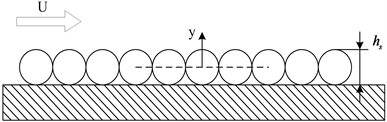Figure 1. Sand roughness model

${u}^{+}=1×\mathrm{ln}\left[{y}^{+}/\left(1+0.3×{k}^{+}\right)\right]/k+C$ (4)

${k}^{+}={y}_{R}×\rho ×{u}^{+}/\mu$ (5)

4. 模型的建立与求解

4.1. 模型建立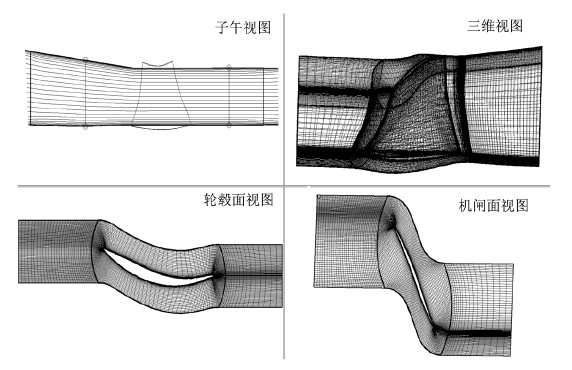Figure 2. View of blade profile

4.2. 仿真计算

5. 仿真结果与分析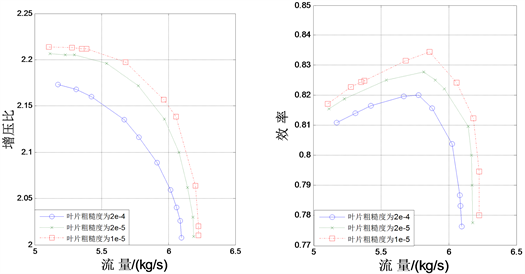Figure 3. The effect of different roughness on compressor characteristics at 100% rotating speed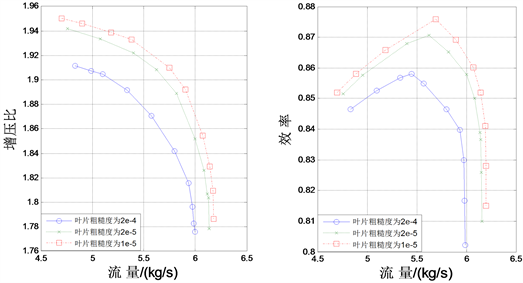Figure 4. The effect of different roughness on compressor characteristics at 90% rotating speed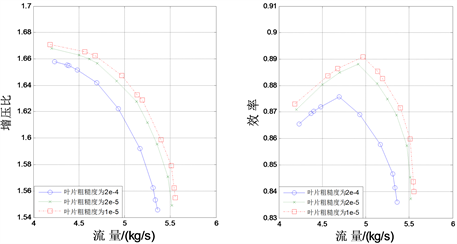Figure 5. The effect of different roughness on compressor characteristics at 80% rotating speed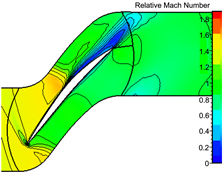(a) ks = 2e−4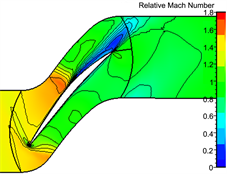(b) ks = 2e−5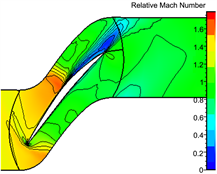(c) ks = 1e−5

Figure 6. The influence of different roughness on shock wave on blade surface when rotating speed is 100%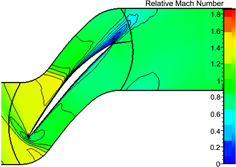(a) ks = 2e−4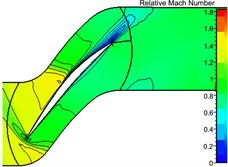(b) ks = 2e−5(c) ks = 1e−5

Figure 7. The influence of different roughness on the shock wave on blade surface when rotating speed is 90%(a) ks = 2e−4(b) ks = 2e−5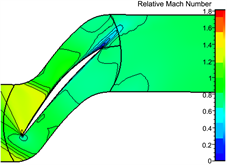(c) ks = 1e−5

Figure 8. The influence of different roughness on the shock wave on blade surface when rotating speed is 80%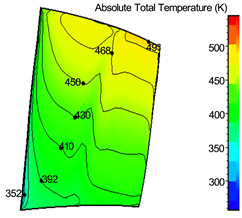(a) ks = 2e−4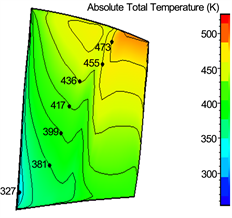(b) ks = 2e−5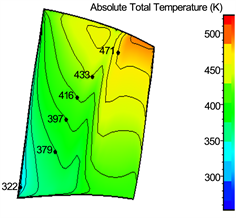(c) ks = 1e−5

Figure 9. The influence of different roughness on the total temperature on blade surface when rotating speed is 100%(a) ks = 2e−4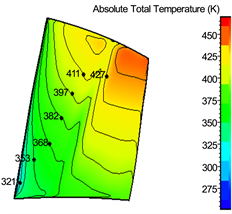(b) ks = 2e−5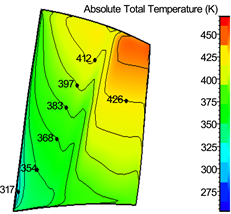(c) ks = 1e−5

Figure 10. The influence of different roughness on the total temperature on blade surface when rotating speed is 90%(a) ks = 2e−4(b) ks = 2e−5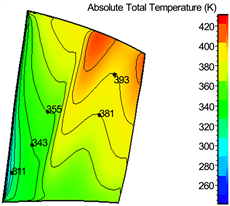(c) ks = 1e−5

Figure 11. The influence of different roughness on the total temperature on blade surface when rotating speed is 80%

6. 结论

1) 叶片表面粗糙度的降低，这将导致压气机增压比和效率显著提高，扩大了压气机稳定工作范围，改善压气机总体工作特性，提高了压气机自身做功的能力，减少了流动损失。

2) 随着叶片表面粗糙度的降低，吸力面侧激波的位置向叶片出口方向逐渐移动，且强度减小明显，进一步使得叶片的尾迹区范围减小。

3) 随着叶片表面粗糙度的降低，叶片表面总温有不同程度的降低。当叶片粗糙度降低后，叶片表面总温的降低幅度从叶片底部到叶片顶部依次减小，随着转速降低依次减小。

Study on the Influence of Blade Roughness on Compressor Performance[J]. 应用物理, 2019, 09(08): 365-372. https://doi.org/10.12677/APP.2019.98043

1. 1. 刘大响. 航空发动机[M]. 北京: 航空工业出版社, 2008.

2. 2. 孙护国. 涡轴发动机清洗技术及其发展[C]//中国航空学会. 第十六届全国直升机年会论文集. 北京: 北京航空航天大学出版社, 2008.

3. 3. Back, S.C., Sohn, J.H. and Song, S.J. (2010) Impact of Surface Roughness on Compressor Cascade Performance. Journal of Fluids Engi-neering, 132, Article ID: 064502.

4. 4. Millsaps, K.T. (2012) Effects of Reynolds Number and Surface Roughness Magnitude and Location on Compressor Cascade Performance. Journal of Turbomachinery, 134, Article ID: 051013. https://doi.org/10.1115/1.4003821

5. 5. Suder, K.L., Chima, R.V., Strazisar, A.J. and Roberts, W.B. (1995) The Effect of Adding Roughness and Thickness to a Transonic Axial Compressor Rotor. ASME Journal of Turbomachinery, 117, 491-505. https://doi.org/10.1115/1.2836561

6. 6. 李本威, 李冬, 李姜华, 等. 单级压气机性能衰退定量研究[J]. 航空动力学报, 2010, 25(7): 1588-1594.

7. 7. 李钊, 李本威, 王东艺, 等. 压气机性能参数对积垢敏感性分析[J]. 航空计算技术, 2011, 41(6): 41-44+48.

8. 8. 郑贇, 田晓, 杨慧. 跨声速风扇叶片变形对气动性能的影响[J]. 航空动力学报, 2011, 26(7): 1621-1627.

9. 9. 陈绍文, 张辰, 石慧, 等. 轴流压气机污垢沉积影响的数值研究[J]. 推进技术, 2012, 33(3): 377-383.

10. 10. 尹松, 金东海, 朱芳, 等. 湍流模型对压气机数值模拟精度的影响[J]. 航空动力学报, 2010, 25(12): 2683-2689.

11. 11. 颜青钦. 涡扇发动机风扇性能衰退机理及其影响规律研究[D]: [硕士学位论文]. 烟台: 海军航空工程学院, 2012.

12. 12. 李绍斌, 苏杰先, 冯国泰, 等. 动静叶片干扰下跨声压气机级性能与叶片型面静压研究[J]. 航空动力学报, 2007, 22(7): 1153-1160.

13. 13. 李本威, 李冬, 沈伟, 等. 涡轮叶片粗糙度对其性能衰退的影响研究[J]. 航空计算技术, 2009, 39(5): 26-29+34.